# 2020 Full-time Interview CCJ’s Preparation (1): Commonly Asked C++ Interview Questions

## 1: What are the differences between C and C++?

• 1) C++ is a kind of superset of C, most of C programs except few exceptions work in C++ as well.

• 2) C is a procedural programming language, but C++ supports both procedural and Object Oriented Programming (OOP).

• 3) Since C++ supports object oriented programming, it supports features like:

• templates,

• inheritance,

• virtual functions,

• friend functions.

• These features are absent in C.

• 4) C++ supports exception handling at language level, in C exception handling is done in traditional if-else style.
5) C++ supports references, C doesn’t.
6) In C, scanf() and printf() are mainly used input/output. C++ mainly uses streams to perform input and output operations. cin is standard input stream and cout is standard output stream.

## 2. What are the differences between references and pointers?

### Their similarities:

• Both references and pointers can be used to change local variables of one function inside another function.

• Both of them can also be used to save copying of big objects when passed as arguments to functions or returned from functions, to get efficiency gain.

### Their differences:

• References are less powerful than pointers:

• 1) Once a reference is created, it cannot be later made to reference another object; it cannot be reseated. This is often done with pointers.

• 2) References cannot be NULL. Pointers are often made NULL to indicate that they are not pointing to any valid thing.

• 3) A reference must be initialized when declared. There is no such restriction with pointers

• Due to the above limitations, references in C++ cannot be used for implementing data structures like Linked List, Tree, etc. In Java, references don’t have above restrictions, and can be used to implement all data structures. References being more powerful in Java, is the main reason Java doesn’t need pointers.

• References are safer and easier to use:

• 1) Safer: Since references must be initialized, wild references like wild pointers are unlikely to exist. It is still possible to have references that don’t refer to a valid location (See questions 5 and 6 in the below exercise )

• 2) Easier to use: References don’t need dereferencing operator to access the value. They can be used like normal variables. ‘&’ operator is needed only at the time of declaration. Also, members of an object reference can be accessed with dot operator (‘.’), unlike pointers where arrow operator (->) is needed to access members.

## 3. What are virtual functions – Write an example?

A virtual function is a member function which is declared in the base class using the keyword virtual and is re-defined (overridden) by the derived class.

Virtual functions are used with inheritance, they are called according to the type of object pointed or referred, not according to the type of pointer or reference. In other words, virtual functions are resolved late, at runtime. Virtual keyword is used to make a function virtual.

Following things are necessary to write a C++ program with runtime polymorphism (use of virtual functions, i.e., 多态性)

• 1) A base class and a derived class.

• 2) A function with same name in base class and derived class.

• 3) A pointer or reference of base class type pointing or referring to an object of derived class.

For example, in the following program bp is a pointer of type Base, but a call to bp->show() calls show() function of Derived class, because bp points to an object of Derived class.

#include<iostream>
using namespace std;

class Base {
public:
virtual void show() { cout<<" In Base \n"; }
};

class Derived: public Base {
public:
void show() { cout<<"In Derived \n"; }
};

int main(void) {
Base *bp = new Derived;
bp->show(); // RUN-TIME POLYMORPHISM
return 0;
}


• Output: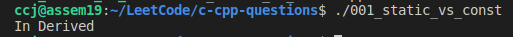## 4. What is this pointer?

The ‘this’ pointer is passed as a hidden argument to all nonstatic member function calls and is available as a local variable within the body of all nonstatic functions.

• ‘this’ pointer is a constant pointer that holds the memory address of the current object.

• ‘this’ pointer is NOT available in static member functions as static member functions can be called without any object (with class name).

• Can we do delete "this"? Ideally delete operator should not be used for "this" pointer. However, if used, then following points must be considered.

• 1) delete operator works only for objects allocated using operator new. If the object is created using new, then we can do delete this, otherwise behavior is undefined.

• 2) Once delete this is done, any member of the deleted object should not be accessed after deletion.

• 3) The best thing is to NOT do delete this at all.

class A {
public:
void fun(){
cout << "try to run 'delete this'\n";
delete this;
}
};

int main() {
/* Following is Valid */
A *ptr = new A;
ptr->fun();
ptr = NULL; // make ptr=NULL to make sure that things are not accessed using ptr;

/* And following is Invalid: Undefined Behavior */
A a;
a.fun();
getchar();
return 0;
}

• To compile using g++: g++ 001_static_vs_const.cpp -o 001_static_vs_const

• Output: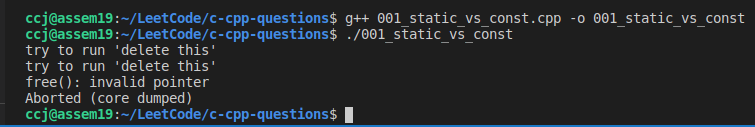## 5. What exactly does an #if 0 … #endif block do?

#if 0
//Code goes here
#endif


#if is a preprocessor command, which gets evaluated before the actual compilation step. The code inside that block doesn’t appear in the compiled binary.

It’s often used for temporarily removing segments of code with the intention of turning them back on later.

## 6. C++的数据: Variables, Literals and Constants

In this section, we will learn about variables, literals, and constants in C++ with the help of examples.

C++的数据包括常量(literal)与变量(variable), 常量与变量都具有类型。

### 1) Variable:

There are following basic types of variable in C++: bool, char, int, float, double, void, wchar_t (A wide character type). C++ also allows to define various other types of variables, like Enumeration(enum), Pointer (*), Array, Reference (&), Structures (struct), and Classes (class).

#### a) Variable Definition in C++:

• A variable definition tells the compiler where and how much storage to create for the variable. A variable definition specifies a data type, and contains a list of one or more variables of that type as follows −

int    i, j, k;
int d = 3, f = 5;  // definition and initializing d and f.
float  f, salary;
double d;

• For definition without an initializer: variables with static storage duration are implicitly initialized withNULL (all bytes have the value 0); the initial value of all other variables is undefined.

#### b) Variable Declaration in C++:

• A variable declaration provides assurance to the compiler that there is one variable existing with the given type and name so that compiler proceed for further compilation without needing complete detail about the variable. A variable declaration has its meaning at the time of compilation only, compiler needs actual variable definition at the time of linking of the program.

• A variable declaration is useful when you are using multiple files and you define your variable in one of the files which will be available at the time of linking of the program. You will use extern keyword to declare a variable at any place. Though you can declare a variable multiple times in your C++ program, but it can be defined only once in a file, a function or a block of code. For example -

#include <iostream>
using namespace std;

// Variable declaration:
extern int a, b;
extern int c;
extern float f;

int main () {
// Variable definition:
int a, b;
int c;
float f;

// actual initialization
a = 10;
b = 20;
c = a + b;

cout << c << endl ;

f = 70.0/3.0;
cout << f << endl ;

return 0;
}

• Same concept applies on function declaration where you provide a function name at the time of its declaration and its actual definition can be given anywhere else. For example −

// function declaration
int func(int);

int main(){
// function call
int i = func();
}

// function definition
int func(int a) {
return a;
}


#### c) Variable Scope in C++:

• A scope is a region of the program and broadly speaking there are three places, where variables can be declared −

• Inside a function or a block which is called local variables,

• In the definition of function parameters which is called formal parameters.

• Outside of all functions which is called global variables.

### 2) C++ Constants/Literals:

Constants refer to fixed values that the program may not alter and they are called literals.

Constants can be of any of the basic data types and can be divided into into Integer Numerals, Floating-Point Numerals, Characters, Strings and Boolean Values. Again, constants are treated just like regular variables except that their values cannot be modified after their definition.

#### a) Integer Literals:

• An integer literal can be a decimal, octal, or hexadecimal constant. A prefix specifies the base or radix: 0x or 0X for hexadecimal, 0 for octal, and nothing for decimal.

• An integer literal can also have a suffix that is a combination of U and L, for unsigned and long, respectively. The suffix can be uppercase or lowercase and can be in any order.

• Here are some examples of integer literals −

212         // Legal
215u        // Legal, unsigned int
0xFeeL      // Legal, L for long int
078         // Illegal: 8 is not an octal digit
032UU       // Illegal: cannot repeat a suffix

//Following are other examples of various types of Integer literals;
85         // decimal
0213       // octal
30         // int
30u        // unsigned int
30l        // long
30ul       // unsigned long


#### b) Floating-point Literals:

• A floating-point literal has an integer part, a decimal point, a fractional part, and an exponent part. You can represent floating point literals either in decimal form or exponential form.

• While representing using decimal form, you must include the decimal point, the exponent, or both and while representing using exponential form, you must include the integer part, the fractional part, or both. The signed exponent is introduced by e or E.

• Here are some examples of floating-point literals −

3.14159       // Legal
314159E-5L    // Legal
510E          // Illegal: incomplete exponent
210f          // Illegal: no decimal or exponent
.e55          // Illegal: missing integer or fraction


#### c) Boolean Literals:

• There are two Boolean literals and they are part of standard C++ keywords:

• A value of true representing true.

• A value of false representing false.

• You should not consider the value of true equal to 1 and value of false equal to 0.

#### d) Character Literals:

• Character literals are enclosed in single quotes (单引号). If the literal begins with L (uppercase only), it is a wide character literal (e.g., L’x’) and should be stored in wchar_t type of variable . Otherwise, it is a narrow character literal (e.g., ‘x’) and can be stored in a simple variable of char type.

• A character literal can be a plain character (e.g., ‘x’), an escape sequence (e.g., ‘\t’), or a universal character (e.g., ‘\u02C0’).

• There are certain characters in C++ when they are preceded by a backslash they will have special meaning and they are used to represent like newline (\n) or tab (\t).

#### e) String Literals:

• String literals are enclosed in double quotes(双引号). A string contains characters that are similar to character literals: plain characters, escape sequences, and universal characters.

• You can break a long line into multiple lines using string literals and separate them using whitespaces.

• Here are some examples of string literals. All the three forms are identical strings.

//All the three forms are identical strings.
"hello, dear"

"hello, \
dear"

"hello, " "d" "ear"


#### f) Defining Constants

• There are two simple ways in C++ to define constants:

• Using #define preprocessor. E.g., #define LENGTH 10, #define WIDTH 5 and #define NEWLINE '\n';

• Using const keyword. E.g., const int LENGTH = 10;, const int WIDTH = 5; and const char NEWLINE = '\n';.

- To make a variable constant, simply put the const keyword either before (which is recommend) or after the variable type, like so:

const double gravity { 9.8 }; // preferred use of const before type
int const sidesInSquare { 4 }; // okay, but not preferred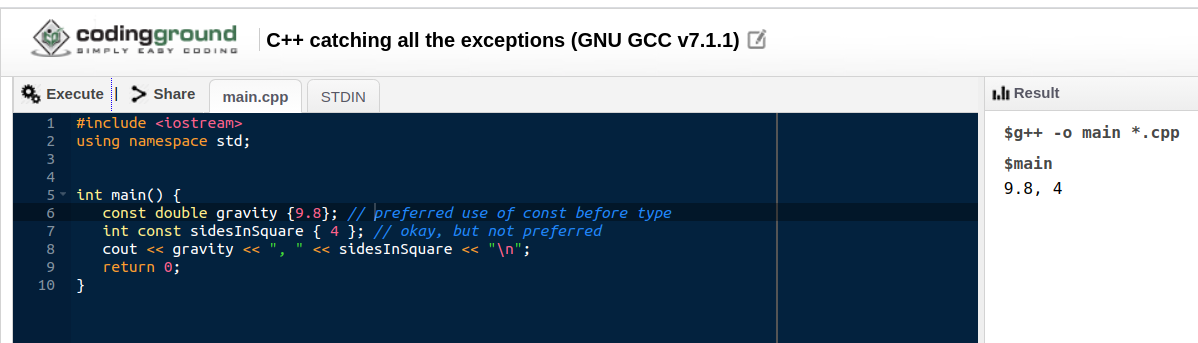• Const variables must be initialized when you define them, and then that value can not be changed via assignment.

• Defining a const variable without initializing it will also cause a compile error

• Note that it is a good programming practice to define constants in CAPITALS.

Live Demo
#include <iostream>
using namespace std;

//#define LENGTH 10
//#define WIDTH  5
//#define NEWLINE '\n'

int main() {
const int  LENGTH = 10;
const int  WIDTH  = 5;
const char NEWLINE = '\n';
int area;

area = LENGTH * WIDTH;
cout << area;
cout << NEWLINE;
return 0;
}


## 7. C++ Modifier Types

The data type modifiers are listed here:

• signed

• unsigned

• long

• short

The modifiers signed, unsigned, long, and short can be applied to integer base types. In addition, signed and unsigned can be applied to char, and long can be applied to double.

The modifiers signed and unsigned can also be used as prefix to long or short modifiers. For example, unsigned long int.

C++ allows a shorthand notation for declaring unsigned, short, or long integers. You can simply use the word unsigned, short, or long, without int. It automatically implies int. For example, the following two statements both declare unsigned integer variables.

unsigned x;
unsigned int y;


## 8. The static Storage Class

• 1) making local variables static:

• The static storage class instructs the compiler to keep a local variable in existence during the life-time of the program instead of creating and destroying it each time it comes into and goes out of scope. Therefore, making local variables static allows them to maintain their values between function calls.

• 2) making global variables static:

• The static modifier may also be applied to global variables. When this is done, it causes that variable’s scope to be restricted to the file in which it is declared.

• 3) making class data member static:

• In C++, when static is used on a class data member, it causes only one copy of that member to be shared by all objects of its class.

#include <iostream>

// Function declaration
void func(void);

static int count = 10; /* Global variable */

main() {
while(count--) {
func();
}
return 0;
}

// Function definition
void func( void ) {
static int i = 5; // local static variable
i++;
std::cout << "i is " << i ;
std::cout << " and count is " << count << std::endl;
}

• When the above code is compiled and executed, it produces the following result −

i is 6 and count is 9
i is 7 and count is 8
i is 8 and count is 7
i is 9 and count is 6
i is 10 and count is 5
i is 11 and count is 4
i is 12 and count is 3
i is 13 and count is 2
i is 14 and count is 1
i is 15 and count is 0


## 9. The extern Storage Class

• The extern storage class is used to give a reference of a global variable that is visible to ALL the program files. When you use 'extern' the variable cannot be initialized as all it does is point the variable name at a storage location that has been previously defined.

• When you have multiple files and you define a global variable or function, which will be used in other files also, then extern will be used in another file to give reference of defined variable or function. Just for understanding extern is used to declare a global variable or function in another file.

• The extern modifier is most commonly used when there are two or more files sharing the same global variables or functions as explained below.

• E.g., First File: main.cpp

#include <iostream>
int count ;
extern void write_extern();

main() {
count = 5;
write_extern();
}

• Second File: support.cpp

#include <iostream>
extern int count;
void write_extern(void) {
std::cout << "Count is " << count << std::endl;
}

• Here, extern keyword is being used to declare count in another file. Now compile these two files as follows −

$g++ main.cpp support.cpp -o my_exam1  This will produce my_exam1 executable program, try to execute it and check the result as follows − $./my_exam1
>>> 5


## 10. In C: What is the difference between ++i and i++?

• ++i will increment the value of i, and then return the incremented value.

 i = 1;
j = ++i;
(i is 2, j is 2)

• i++ will increment the value of i, but return the original value that i held before being incremented.

 i = 1;
j = i++;
(i is 2, j is 1)

• So basically ++i returns the value after it is incremented, while i++ return the value before it is incremented. At the end, in both cases the i will have its value incremented.

• For a for loop, either works. ++i seems more common, perhaps because that is what is used in K&R.

• In any case, follow the guideline "prefer ++i over i++" and you won’t go wrong.

## 11. Bitwise Operator

 C++ Python Note Examples & & Binary AND Operator x & y: Does a “bitwise and”. Each bit of the output is 1 if the corresponding bit of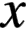AND of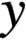is 1, otherwise it’s 0. | | Binary OR Operator x | y: Does a “bitwise or”. Each bit of the output is 0 if the corresponding bit ofAND ofis 0, otherwise it’s 1 ^ ^ Binary XOR Operator x ^ y: Does a “bitwise exclusive or”. Each bit of the output is the same as the corresponding bit in x if that bit in y is 0, and it’s the complement of the bit in x if that bit in y is 1 ~ ~ Binary Ones Complement Operator ~ x: Returns the complement of x. i.e., the number you get by switching each 1 for a 0 and each 0 for a 1. This is the same as -x - 1 << << Binary Left Shift Operator. x << y: Returnswith the bits shifted to the left byplaces (and new bits on the right-hand-side are zeros). This is the same as multiplyingby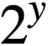>> >> Binary Right Shift Operator. x >> y: Returnswith the bits shifted to the right byplaces. This is the same as //’ingby## 12. Logical Operators

 C++ Python Note Examples && and Called Logical AND operator x && y(C++) or x and y(Python): Returns True if both statements are true || or Called Logical OR operator x || y(C++) or x or y(Python): Returns True if one of the statements is true ! not Called Logical NOT operator !x(C++) or not x(Python): Reverse the result, returns False if the result is true

## 13. Misc Operators

The following table lists some other operators that C++ supports.

 Sr.No Operator & Description sizeof sizeof operator returns the size of a variable. For example, sizeof(a), where ‘a’ is integer, and will return 4. Condition ? X : Y Conditional operator (?). If Condition is true then it returns value of X otherwise returns value of Y. , Comma operator Comma operator causes a sequence of operations to be performed. The value of the entire comma expression is the value of the last expression of the comma-separated list. . (dot) and -> (arrow) Member operators are used to reference individual members of classes, structures, and unions. Cast, e.g., int(x) Casting operators convert one data type to another. For example, int(2.2000) would return 2. This syntax works in both C++ and Python. & Pointer operator Pointer operator & returns the address of a variable. For example &a; will give actual address of the variable. * Pointer operator * is pointer to a variable. For example *var; will pointer to a variable var.

## 14. Loop Types in C++ and Python

### 1) while loop:

• With the break statement we can stop the loop even if the while condition is true:

• With the continue statement we can stop the current iteration, and continue with the next:

• C++ Syntax : Here, statement(s) may be a single statement or a block of statements. The condition may be any expression, and true is any non-zero value. The loop iterates while the condition is true. When the condition becomes false, program control passes to the line immediately following the loop.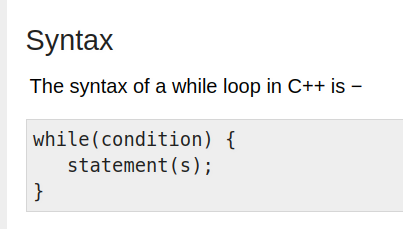• Python Syntax: note with the else statement we can run a block of code once when the condition no longer is true: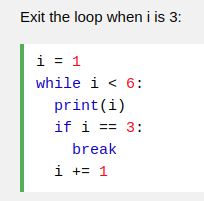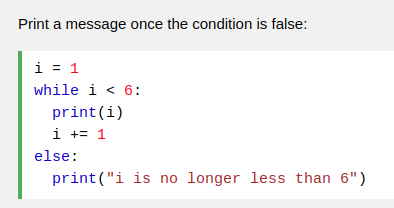### 2) for loop:

• C++ Syntax: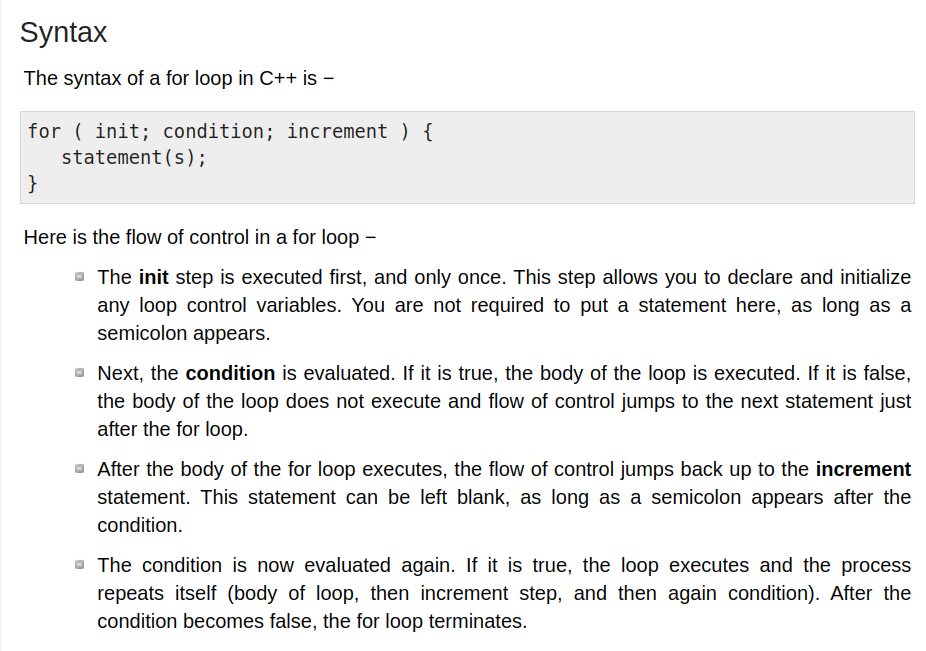// for loop execution
for( int i = 10; i < 20; ++i){
cout << "value of i: " << i << endl;
}

• Python Syntax:

for x in range(6):
print(x)


### 3) do … while loop in C++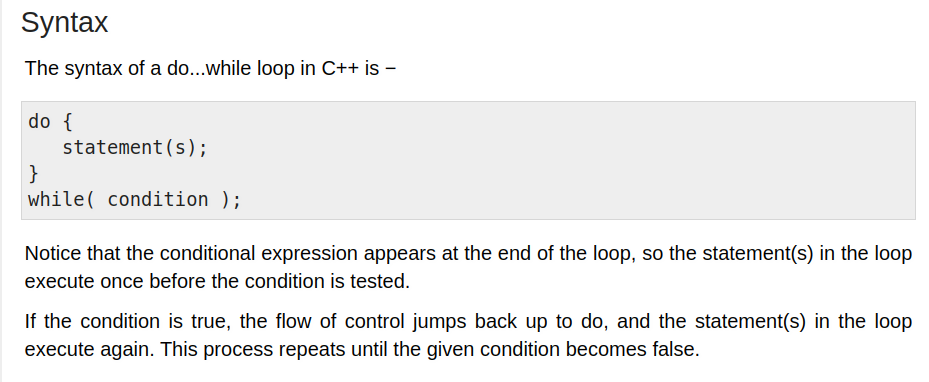## 15. Loop Control Statements in C++ and Python

C++ supports the following control statements.

• break statement (C++ and Python): Terminates the loop or switch statement and transfers execution to the statement immediately following the loop or switch.

• continue statement (C++ and Python): Causes the loop to skip the remainder of its body and immediately retest its condition prior to reiterating.

• goto statement (C++): Transfers control to the labeled statement. Though it is not advised to use goto statement in your program.

## 16. C++ Pointers

### 1) What are Pointers?

• As you know every variable is a memory location and every memory location has its address defined which can be accessed using ampersand (&) operator which denotes an address in memory.

int  var1;
char var2;

cout << "Address of var1 variable: " << &var1 << endl;
// Address of var1 variable: 0xbfebd5c0

cout << "Address of var2 variable: " << &var2 << endl;
// Address of var2 variable: 0xbfebd5b6

• A pointer is a variable whose value is the address of another variable. Like any variable or constant, you must declare a pointer before you can work with it. The general form of a pointer variable declaration is − type *var-name;, e.g.,

int    *ip = NULL;    // pointer to an integer
double *dp;    // pointer to a double
float  *fp;    // pointer to a float
char   *ch     // pointer to character

• The NULL pointer is a constant with a value of zero defined in several standard libraries, including iostream.

• To check for a null pointer you can use an if statement as follows −

• if(ptr) succeeds if p is not null

• if(!ptr) succeeds if p is null

### 2) Pointers vs Arrays

• In fact, pointers and arrays are interchangeable in many cases. For example, a pointer that points to the beginning of an array can access that array by using either pointer arithmetic or array-style indexing.

int  var[MAX] = {10, 100, 200};
int  *ptr;

// let us have array address in pointer.
ptr = var;

for (int i = 0; i < MAX; i++) {
cout << "Address of var[" << i << "] = " << ptr << endl;

cout << "Value of var[" << i << "] = " << *ptr << endl;

// point to the next location
ptr++;
}


When the above code is compiled and executed, it produces result something as follows −

Address of var = 0xbfa088b0
Value of var = 10
Value of var = 100
Value of var = 200


#### b) pointers and arrays are not completely interchangeable

• However, pointers and arrays are not completely interchangeable. For example, consider the following program −

#include <iostream>

using namespace std;
const int MAX = 3;

int main () {
int  var[MAX] = {10, 100, 200};

for (int i = 0; i < MAX; i++) {
*var = i;    // This is a correct syntax
var++;       // This is incorrect.
}

return 0;
}

• It is perfectly acceptable to apply the pointer operator * to var but it is ILLEGAL to modify var value. The reason for this is that var is a constant that points to the beginning of an array and can not be used as l-value(i.e., left side value wrt assignment =).

• Because an array name generates a pointer constant, it can still be used in pointer-style expressions, as long as it is not modified. For example, the following is a valid statement that assigns var the value 500 −

*(var + 2) = 500;

• Above statement is valid and will compile successfully because var itself is not changed.

## 17. C++ References

### 1) What are References?

A reference variable is an alias, that is, another name for an already existing variable. Once a reference is initialized with a variable, either the variable name or the reference name may be used to refer to the variable.

### 2) References vs Pointers

References are often confused with pointers but three major differences between references and pointers are −

• You CANNOT have NULL references. You must always be able to assume that a reference is connected to a legitimate piece of storage.

• Once a reference is initialized to an object, it cannot be changed to refer to another object. Pointers can be pointed to another object at any time.

• A reference must be initialized when it is created. Pointers can be initialized at any time.

### 3) Creating References in C++

• Think of a variable name as a label attached to the variable’s location in memory. You can then think of a reference as a second label attached to that memory location. Therefore, you can access the contents of the variable through either the original variable name or the reference. For example, suppose we have the following example −

int i = 17;

We can declare reference variables for i as follows.

int& r = i;

Read the & in these declarations as reference. Thus, read the first declaration as "r is an integer reference initialized to i" and read the second declaration as "s is a double reference initialized to d.". Following example makes use of references on int and double −

Live Demo
#include <iostream>

using namespace std;

int main () {
// declare simple variables
int    i = 5;
double d = 11.7;

// declare reference variables
int&    r = i;
double& s = d;

cout << "Value of i : " << i << endl;
cout << "Value of i reference : " << r  << endl;

cout << "Value of d : " << d << endl;
cout << "Value of d reference : " << s  << endl;

return 0;
}


When the above code is compiled together and executed, it produces the following result −

Value of i : 5
Value of i reference : 5
Value of d : 11.7
Value of d reference : 11.7


### 4) References for function argument lists

References are usually used for function argument lists and function return values. So following are two important subjects related to C++ references which should be clear to a C++ programmer −

#### a) References as Parameters

• C++ supports passing references as function parameter more safely than parameters.

• We have discussed how we implement call by reference concept using pointers. Here is another example of call by reference which makes use of C++ reference −

#include <iostream>
using namespace std;

// function declaration
void swap(int& x, int& y);

int main () {
// local variable declaration:
int a = 100;
int b = 200;

cout << "Before swap, value of a :" << a << endl;
cout << "Before swap, value of b :" << b << endl;

/* calling a function to swap the values.*/
swap(a, b);

cout << "After swap, value of a :" << a << endl;
cout << "After swap, value of b :" << b << endl;

return 0;
}

// function definition to swap the values.
void swap(int& x, int& y) {

int temp;
temp = x; /* save the value at address x */
x = y;    /* put y into x */
y = temp; /* put x into y */

return;
}


#### b) Reference as Return Value

• You can return reference from a C++ function like any other data type.

• A C++ program can be made easier to read and maintain by using references rather than pointers.

• A C++ function can return a reference in a similar way as it returns a pointer.

• When a function returns a reference, it returns an implicit pointer to its return value. This way, a function can be used on the left side of an assignment statement. For example, consider this simple program −

#include <iostream>
#include <ctime>

using namespace std;

double vals[] = {10.1, 12.6, 33.1, 24.1, 50.0};

double& setValues( int i ) {
return vals[i];   // return a reference to the ith element
}

// main function to call above defined function.
int main () {

cout << "Value before change" << endl;
for ( int i = 0; i < 5; i++ ) {
cout << "vals[" << i << "] = ";
cout << vals[i] << endl;
}

setValues(1) = 20.23; // change 2nd element
setValues(3) = 70.8;  // change 4th element

cout << "Value after change" << endl;
for ( int i = 0; i < 5; i++ ) {
cout << "vals[" << i << "] = ";
cout << vals[i] << endl;
}
return 0;
}

• When the above code is compiled together and executed, it produces the following result:

Value before change
vals = 10.1
vals = 12.6
vals = 33.1
vals = 24.1
vals = 50
Value after change
vals = 10.1
vals = 20.23
vals = 33.1
vals = 70.8
vals = 50

• When returning a reference, be careful that the object being referred to does not go out of scope. So it is not legal to return a reference to local var. But you can always return a reference on a static variable.

int& func() {
int q;
//! return q; // Compile time error
static int x;
return x;     // Safe, x lives outside this scope
}


## 18. C++ Functions

A function is a group of statements that together perform a task. Every C++ program has at least one function, which is main(), and all the most trivial programs can define additional functions.

### 1) Defining a Function

• Parameters − A parameter is like a placeholder. When a function is invoked, you pass a value to the parameter. This value is referred to as actual parameter (opposed to the formal parameter below ) or argument. The parameter list refers to the type, order, and the number of the parameters of a function. Parameters are optional; that is, a function may contain no parameters.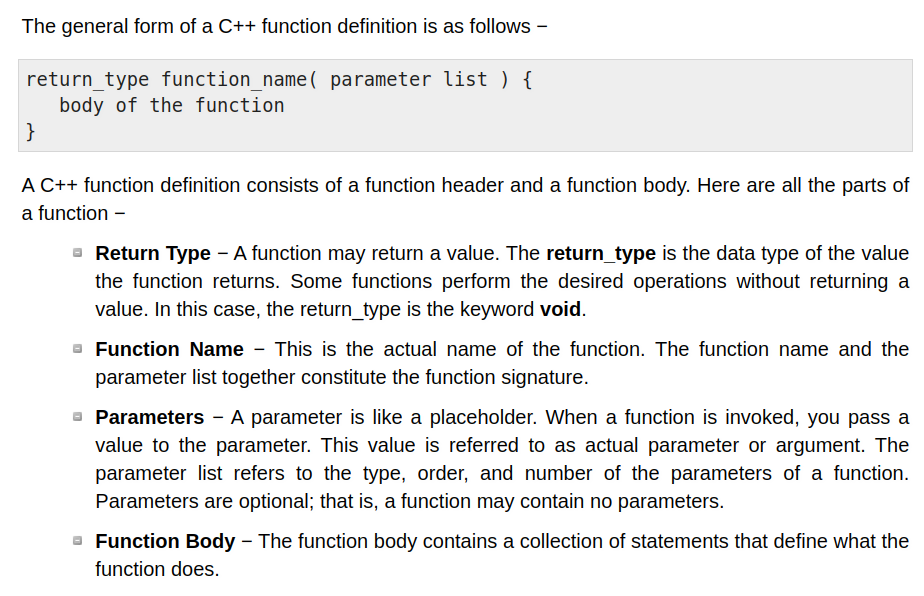### 2) Function Declarations

• A function declaration tells the compiler about a function name and how to call the function. The actual body of the function can be defined separately.

• A function declaration has the following parts −

• return_type function_name( parameter list );

• e.g., int max(int num1, int num2);

• Parameter names are not important in function declaration only their type is required, so following is also valid declaration: int max(int, int);

• Function declaration is required when you define a function in one source file and you call that function in another file. In such case, you should declare the function at the top of the file calling the function.

### 3) Function Arguments

• If a function is to use arguments, it must declare variables that accept the values of the arguments. These variables are called the formal parameters of the function.

• The formal parameters behave like other local variables inside the function and are created upon entry into the function and destroyed upon exit.

### 4) Calling a Function in C++

• When a program calls a function, program control is transferred to the called function. A called function performs defined task and when it’s return statement is executed or when its function-ending closing brace is reached, it returns program control back to the main program.

• To call a function, you simply need to pass the required parameters along with function name, and if function returns a value, then you can store returned value.

• While calling a function, there are several ways that arguments can be passed to a function:

#### a) call by value:

The call by value method of passing arguments to a function copies the actual value of an argument into the formal parameter of the function. In this case, changes made to the parameter inside the function have no effect on the argument.
By default, C++ uses call by value to pass arguments. In general, this means that code within a function cannot alter the arguments used to call the function.

#### b) call by pointer:

The call by pointer method of passing arguments to a function copies the address of an argument into the formal parameter. Inside the function, the address is used to access the actual argument used in the call. This means that changes made to the parameter affect the passed argument.

To pass the value by pointer, argument pointers are passed to the functions just like any other value. So accordingly you need to declare the function parameters as pointer types as in the following function swap(), which exchanges the values of the two integer variables pointed to by its arguments.

// function definition to swap the values.
void swap(int *x, int *y) {
int temp;
temp = *x; /* save the value at address x */
*x = *y; /* put y into x */
*y = temp; /* put x into y */
return;
}


#### c) call by reference:

The call by reference method of passing arguments to a function copies the reference of an argument into the formal parameter. Inside the function, the reference is used to access the actual argument used in the call. This means that changes made to the parameter affect the passed argument.

To pass the value by reference, argument reference is passed to the functions just like any other value. So accordingly you need to declare the function parameters as reference types as in the following function swap(), which exchanges the values of the two integer variables pointed to by its arguments.

// function definition to swap the values.
void swap(int &x, int &y) {
int temp;
temp = x; /* save the value at address x */
x = y;    /* put y into x */
y = temp; /* put x into y */

return;
}


## 19: C++ Data Structures: struct

C/C++ arrays allow you to define variables that combine several data items of the same kind, but structure is another user defined data type which allows you to combine data items of different kinds.

### 1) Defining a Structure

To define a structure, you must use the struct statement. The struct statement defines a new data type, with more than one member, for your program. The format of the struct statement is this −

struct [structure tag] {
member definition;
member definition;
...
member definition;
} [one or more structure variables];


The structure tag is optional and each member definition is a normal variable definition, such as int i; or float f; or any other valid variable definition. At the end of the structure’s definition, before the final semicolon, you can specify one or more structure variables but it is optional. Here is the way you would declare the Book structure −

struct Books {
char  title;
char  author;
char  subject;
int   book_id;
} book;


### 2) Accessing Structure Members

To access any member of a structure, we use the member access operator (.). The member access operator is coded as a period between the structure variable name and the structure member that we wish to access. You would use struct keyword to define variables of structure type. Following is the example to explain usage of structure −

struct Books {
char  title;
char  author;
char  subject;
int   book_id;
};

struct Books Book1; // Declare Book1 of type Book
// book 1 specification
strcpy( Book1.title, "Learn C++ Programming");
strcpy( Book1.author, "Chand Miyan");
strcpy( Book1.subject, "C++ Programming");
Book1.book_id = 6495407;


### 3) Structures as Function Arguments

You can pass a structure as a function argument in very similar way as you pass any other variable or pointer. You would access structure variables in the similar way as you have accessed in the above example −

void printBook( struct Books book ) {
cout << "Book title : " << book.title <<endl;
cout << "Book author : " << book.author <<endl;
cout << "Book subject : " << book.subject <<endl;
cout << "Book id : " << book.book_id <<endl;
}

printBook( Book1 );


### 4) Pointers to Structures

You can define pointers to structures in very similar way as you define pointer to any other variable as follows −

• struct Books *struct_pointer;

Now, you can store the address of a structure variable in the above defined pointer variable. To find the address of a structure variable, place the & operator before the structure’s name as follows −

• struct_pointer = &Book1;

To access the members of a structure using a pointer to that structure, you must use the -> operator as follows −

• struct_pointer->title;

### 5) The typedef Keyword

There is an easier way to define structs or you could “alias” types you create. For example −

typedef struct {
char  title;
char  author;
char  subject;
int   book_id;
} Books;


Now, you can use Books directly to define variables of Books type without using struct keyword. Following is the example −

• Books Book1, Book2;

You can use typedef keyword for non-structs as well as follows −

• typedef long int *pint32;

• pint32 x, y, z;

Then x, y and z are all pointers to long ints.

## 20. C++ Classes and Objects

• The main purpose of C++ programming is to add object orientation to the C programming language and classes are the central feature of C++ that supports object-oriented programming and are often called user-defined types.

• The data and functions within a class are called members of the class.

### 1) C++ Class Definitions

A class definition starts with the keyword class followed by the class name; and the class body, enclosed by a pair of curly braces. A class definition must be followed either by a semicolon or a list of declarations. For example, we defined the Box data type using the keyword class as follows −

class Box {
public:
double length;   // Length of a box
double height;   // Height of a box
};


The keyword public determines the access attributes of the members of the class that follows it. A public member can be accessed from outside the class anywhere within the scope of the class object. You can also specify the members of a class as private or protected which we will discuss in a sub-section.

### 2) Define C++ Objects

A class provides the blueprints for objects, so basically an object is created from a class. We declare objects of a class with exactly the same sort of declaration that we declare variables of basic types. Following statements declare two objects of class Box −

Box Box1;          // Declare Box1 of type Box
Box Box2;          // Declare Box2 of type Box


Both of the objects Box1 and Box2 will have their own copy of data members.

### 3) Accessing the Data Members

The public data members of objects of a class can be accessed using the direct member access operator (.).

### 4) C++ Class Member Functions

• Member functions can be defined within the class definition or separately using scope resolution operator (::).

• Defining a member function within the class definition declares the function inline, even if you do not use the inline specifier. So either you can define Volume() function as below −

class Box {
public:
double length;      // Length of a box
double height;      // Height of a box

double getVolume(void) {
return length * breadth * height;
}
};

• If you like, you can define the same function outside the class using the scope resolution operator (::) as follows −

double Box::getVolume(void) {
return length * breadth * height;
}

• Here, only important point is that you would have to use class name just before :: operator. A member function will be called using a dot operator (.) on a object where it will manipulate data related to that object only as follows −

Box myBox;          // Create an object
myBox.getVolume();  // Call member function for the object


### 5) C++ Class Access Modifiers: public, private, and protected

Data hiding is one of the important features of Object Oriented Programming (OOP) which allows preventing the functions of a program to access directly the internal representation of a class type. The access restriction to the class members is specified by the labeled public, private, and protected sections within the class body.

The keywords public, private, and protected are called access specifiers.

A class can have multiple public, protected, or private labeled sections. Each section remains in effect until either another section label or the closing right brace of the class body is seen.

The default access for members and classes is private.

#### a) The public Members:

• A public member is accessible from anywhere outside the class but within a program. You can set and get the value of public variables without any member function.

#### b) The private Members:

• A private member variable or function cannot be accessed, or even viewed from outside the class.

• Only the class and friend functions can access private members.

• By default all the members of a class would be private, for example in the following class width is a private member, which means until you label a member, it will be assumed a private member −

class Box {
double width;

public:
double length;
void setWidth( double wid );
double getWidth( void );
};


Practically, we define data in private section and related functions in public section so that they can be called from outside of the class as shown in the following program.

#include <iostream>

using namespace std;

class Box {
public:
double length;
void setWidth( double wid );
double getWidth( void );

private:
double width;
};

// Member functions definitions
double Box::getWidth(void) {
return width ;
}

void Box::setWidth( double wid ) {
width = wid;
}

// Main function for the program
int main() {
Box box;

// set box length without member function
box.length = 10.0; // OK: because length is public
cout << "Length of box : " << box.length <<endl;

// set box width without member function
// box.width = 10.0; // Error: because width is private
box.setWidth(10.0);  // Use member function to set it.
cout << "Width of box : " << box.getWidth() <<endl;

return 0;
}


When the above code is compiled and executed, it produces the following result −

Length of box : 10
Width of box : 10


#### c) The protected Members

A protected member variable or function is very similar to a private member but it provided one additional benefit that they can be accessed in child classes which are called derived classes.

You can check following example where I have derived one child class SmallBox from a parent class Box.

Following example is similar to above example and here width member will be accessible by any member function of its derived class SmallBox.

include <iostream>
using namespace std;

class Box {
protected:
double width;
};

class SmallBox:Box { // SmallBox is the derived class.
public:
void setSmallWidth( double wid );
double getSmallWidth( void );
};

// Member functions of child class
double SmallBox::getSmallWidth(void) {
return width ;
}

void SmallBox::setSmallWidth( double wid ) {
width = wid;
}

// Main function for the program
int main() {
SmallBox box;

// set box width using member function
box.setSmallWidth(5.0);
cout << "Width of box : "<< box.getSmallWidth() << endl;

return 0;
}


• When the above code is compiled and executed, it produces the following result −

Width of box : 5


### 6) The Class Constructor and Destructor

#### a) Class Constructor:

• A class constructor is a special member function of a class that is executed whenever we create new objects of that class.

• A constructor will have exact same name as the class and it does not have any return type at all, not even void. Constructors can be very useful for setting initial values for certain member variables.

• A default constructor does not have any parameter.

• Parameterized Constructor: But if you need, a constructor can have parameters. This helps you to assign initial value to an object at the time of its creation.

#include <iostream>

using namespace std;
class Line {
public:
void setLength( double len );
double getLength( void );
Line ();// This is a default constructor;
Line(double len);  // This is a parameterized constructor;

private:
double length;
};

// Member functions definitions including constructor
Line::Line(void) {
cout << "Object is being created" << endl;
}

// Member functions definitions including constructor
Line::Line( double len) {
cout << "Object is being created, length = " << len << endl;
length = len;
}
void Line::setLength( double len ) {
length = len;
}
double Line::getLength( void ) {
return length;
}

// Main function for the program
int main() {
Line line(10.0);

// get initially set length.
cout << "Length of line : " << line.getLength() <<endl;

// set line length again
line.setLength(6.0);
cout << "Length of line : " << line.getLength() <<endl;

return 0;
}

• Using Initialization Lists to Initialize Fields:

• In case of parameterized constructor, you can use following syntax to initialize the fields:

Line::Line( double len): length(len) {
cout << "Object is being created, length = " << len << endl;
}


If for a class A, you have multiple fields X, Y, Z, etc., to be initialized, then use can use same syntax and separate the fields by comma as follows −

A::A( double a, double b, double c): X(a), Y(b), Z(c) {
....
}


#### b) Class Destructor:

• A destructor is a special member function of a class that is executed whenever an object of it’s class goes out of scope or whenever the delete expression is applied to a pointer to the object of that class.

• A destructor will have exact same name as the class prefixed with a tilde (~) and it can neither return a value nor can it take any parameters. Destructor can be very useful for releasing resources before coming out of the program like closing files, releasing memories etc.

// Member functions definitions including constructor
Line::Line(void) {
cout << "Object is being created" << endl;
}
Line::~Line(void) {
cout << "Object is being deleted" << endl;
}


#### c) Copy Constructor:

• The copy constructor is a constructor which creates an object by initializing it with an object of the same class, which has been created previously. The copy constructor is used to:

• Initialize one object from another of the same type.

• Copy an object to pass it as an argument to a function.

• Copy an object to return it from a function.

• If a copy constructor is not defined in a class, the compiler itself defines one.

• If the class has pointer variables and has some dynamic memory allocations, then it is a must to have a copy constructor.

• The most common form of copy constructor is shown here −

classname (const classname &obj) {
// body of constructor
}


For example:

Live Demo
#include <iostream>

using namespace std;

class Line {

public:
int getLength( void );
Line( int len );          // simple constructor
Line( const Line &obj);  // copy constructor
~Line();                 // destructor

private:
int *ptr;
};

// Member functions definitions including constructor
Line::Line(int len) {
cout << "Normal constructor allocating ptr" << endl;
// allocate memory for the pointer;
ptr = new int;
*ptr = len;
}

Line::Line(const Line &obj) {
cout << "Copy constructor allocating ptr." << endl;
ptr = new int;
*ptr = *obj.ptr; // copy the value
}

Line::~Line(void) {
cout << "Freeing memory!" << endl;
delete ptr;
}

int Line::getLength( void ) {
return *ptr;
}

void display(Line obj) {
cout << "Length of line : " << obj.getLength() <<endl;
}

void display2(Line & obj) {
cout << "Length of line : " << obj.getLength() <<endl;
}

// Main function for the program
int main() {
cout << "creating object line1" << endl;
Line line1(10);
cout << "\ncreating object line2" << endl;
Line line2 = line1; // This also calls copy constructor

cout << "\ncalling display(line1)" << endl;
display(line1);
cout << "\ncalling display(line2)" << endl;
display(line2);

cout << "\ncalling display2(line2)" << endl;
display2(line2);

return 0;
}

• When the above code is compiled and executed, it produces the following result −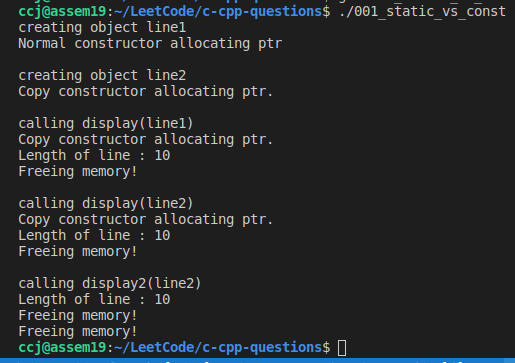• Note:

• The copy constructor is also used to copy an object to pass it as an argument to a function, as shown in calling display(line1);.

• So if we use pass-by-reference for display2, then we can avoid the copy.

### 7) C++ Friend Functions

• A friend function of a class is defined outside that class’ scope but it has the right to access all private and protected members of the class. Even though the prototypes for friend functions appear in the class definition, friends are not member functions.

• A friend can be a function, function template, or member function, or a class or class template, in which case the entire class and all of its members are friends.

• To declare a function as a friend of a class, precede the function prototype in the class definition with keyword friend as follows −

class Box {
double width;

public:
double length;
friend void printWidth( Box box );
void setWidth( double wid );
};


To declare all member functions of class ClassTwo as friends of class ClassOne, place a following declaration in the definition of class ClassOne

friend class ClassTwo;

• Consider the following program −

#include <iostream>

using namespace std;

class Box {
double width;

public:
friend void printWidth( Box box );
void setWidth( double wid );
};

// Member function definition
void Box::setWidth( double wid ) {
width = wid;
}

// Note: printWidth() is not a member function of any class.
void printWidth( Box box ) {
/* Because printWidth() is a friend of Box, it can
directly access any member of this class */
cout << "Width of box : " << box.width <<endl;
}

// Main function for the program
int main() {
Box box;

// set box width without member function
box.setWidth(10.0);

// Use friend function to print the wdith.
printWidth( box );

return 0;
}


When the above code is compiled and executed, it produces the following result −

Width of box : 10


### 8) C++ Inline Functions

• C++ inline function is powerful concept that is commonly used with classes. If a function is inline, the compiler places a copy of the code of that function at each point where the function is called at compile time.

• Any change to an inline function could require all clients of the function to be recompiled because compiler would need to replace all the code once again otherwise it will continue with old functionality.

• To inline a function, place the keyword inline before the function name and define the function before any calls are made to the function. The compiler can ignore the inline qualifier in case defined function is more than a line.

• A function definition in a class definition is an inline function definition, even without the use of the inline specifier.

inline int Max(int x, int y) {
return (x > y)? x : y;
}


### 9) C++ this Pointer

• Every object in C++ has access to its own address through an important pointer called this pointer. The this pointer is an implicit parameter to all member functions. Therefore, inside a member function, this may be used to refer to the invoking object.

• Friend functions do not have a this pointer, because friends are not members of a class. Only member functions have a this pointer.

• Let us try the following example to understand the concept of this pointer −

#include <iostream>

using namespace std;

class Box {
public:
// Constructor definition
Box(double l = 2.0, double b = 2.0, double h = 2.0) {
cout <<"Constructor called." << endl;
length = l;
height = h;
}
double Volume() {
return length * breadth * height;
}
int compare(Box box) {
return this->Volume() > box.Volume();
}

private:
double length;     // Length of a box
double height;     // Height of a box
};

int main(void) {
Box Box1(3.3, 1.2, 1.5);    // Declare box1
Box Box2(8.5, 6.0, 2.0);    // Declare box2

if(Box1.compare(Box2)) {
cout << "Box2 is smaller than Box1" <<endl;
} else {
cout << "Box2 is equal to or larger than Box1" <<endl;
}

return 0;
}


When the above code is compiled and executed, it produces the following result −

Constructor called.
Constructor called.
Box2 is equal to or larger than Box1


### 10) Static Members of a C++ Class

#### a) Static Data Members:

• We can define class members static using static keyword. When we declare a member of a class as static it means no matter how many objects of the class are created, there is only one copy of the static member.

• A static member is shared by all objects of the class. All static data is initialized to zero when the first object is created, if no other initialization is present. We can’t put it in the class definition but it can be initialized outside the class as done in the following example by redeclaring the static variable, using the scope resolution operator :: to identify which class it belongs to.

• Let us try the following example to understand the concept of static data members −

#include <iostream>

using namespace std;

class Box {
public:
static int objectCount;

// Constructor definition
Box(double l = 2.0, double b = 2.0, double h = 2.0) {
cout <<"Constructor called." << endl;
length = l;
height = h;

// Increase every time object is created
objectCount++;
}
double Volume() {
return length * breadth * height;
}

private:
double length;     // Length of a box
double height;     // Height of a box
};

// Initialize static member of class Box
int Box::objectCount = 0;

int main(void) {
Box Box1(3.3, 1.2, 1.5);    // Declare box1
Box Box2(8.5, 6.0, 2.0);    // Declare box2

// Print total number of objects.
cout << "Total objects: " << Box::objectCount << endl;
// Or we can access the static data via an object;
cout << "Total objects: " << Box2.objectCount << endl;
return 0;
}


When the above code is compiled and executed, it produces the following result −

Constructor called.
Constructor called.
Total objects: 2

• Please note static data members can be accessed using either the class name and the scope resolution operator ::, or the object, as shown above:

// Print total number of objects.
cout << "Total objects: " << Box::objectCount << endl;
// Or we can access the static data via an object;
cout << "Total objects: " << Box2.objectCount << endl;


#### b) Static Function Members:

• By declaring a function member as static, you make it independent of any particular object of the class.

• A static member function can be called even if no objects of the class exist and the static functions are accessed using only the class name and the scope resolution operator ::. Please note static data members can be accessed using either the class name and the scope resolution operator ::, or the object.

• A static member function can only access

• i) static data member,

• ii) other static member functions and

• iii) any other functions from outside the class.

• Static member functions have a class scope and they do not have access to the this pointer of the class. You could use a static member function to determine whether some objects of the class have been created or not.

• Let us try the following example to understand the concept of static function members −

#include <iostream>

using namespace std;

class Box {
public:
static int objectCount;

// Constructor definition
Box(double l = 2.0, double b = 2.0, double h = 2.0) {
cout <<"Constructor called." << endl;
length = l;
height = h;

// Increase every time object is created
objectCount++;
}
double Volume() {
return length * breadth * height;
}
// static member function
static int getCount() {
return objectCount;
}

private:
double length;     // Length of a box
double height;     // Height of a box
};

// Initialize static member of class Box
int Box::objectCount = 0;

int main(void) {
// Print total number of objects before creating object.
cout << "Inital Stage Count: " << Box::getCount() << endl;

Box Box1(3.3, 1.2, 1.5);    // Declare box1
Box Box2(8.5, 6.0, 2.0);    // Declare box2

// Print total number of objects after creating object.
cout << "Final Stage Count: " << Box::getCount() << endl;

return 0;
}

• When the above code is compiled and executed, it produces the following result −

Inital Stage Count: 0
Constructor called.
Constructor called.
Final Stage Count: 2


## 21. C++ Inheritance

One of the most important concepts in object-oriented programming is that of inheritance. Inheritance allows us to define a class in terms of another class, which makes it easier to create and maintain an application. This also provides an opportunity to reuse the code functionality and fast implementation time.

When creating a class, instead of writing completely new data members and member functions, the programmer can designate that the new class should inherit the members of an existing class. This existing class is called the base class, and the new class is referred to as the derived class.

The idea of inheritance implements is a relationship. For example, mammal IS-A animal, dog IS-A mammal hence dog IS-A animal as well and so on.

### 1) Base and Derived Classes

A class can be derived from more than one classes, which means it can inherit data and functions from multiple base classes. To define a derived class, we use a class derivation list to specify the base class(es). A class derivation list names one or more base classes and has the form −

class derived-class: access-specifier base-class

• Where access-specifier is one of public, protected, or private,

• and base-class is the name of a previously defined class.

• If the access-specifier is not used, then it is private by default.

Consider a base class Shape and its derived class Rectangle as follows −

#include <iostream>

using namespace std;

// Base class
class Shape {
public:
void setWidth(int w) {
width = w;
}
void setHeight(int h) {
height = h;
}

protected:
int width;
int height;
};

// Derived class
class Rectangle: public Shape {
public:
int getArea() {
return (width * height);
}
};

int main(void) {
Rectangle Rect;

Rect.setWidth(5);
Rect.setHeight(7);

// Print the area of the object.
cout << "Total area: " << Rect.getArea() << endl;

return 0;
}

• When the above code is compiled and executed, it produces the following result −

Total area: 35


### b) Access Control and Inheritance

• A derived class can access all the non-private members of its base class. Thus base-class members that should not be accessible to the member functions of derived classes should be declared private in the base class.

• We can summarize the different access types according to - who can access them in the following way −

 Access public protected private Same class yes yes yes Derived classes yes yes no Outside classes yes no no
• A derived class inherits all base class methods with the following exceptions −

• Constructors, destructors and copy constructors of the base class.

• Overloaded operators of the base class.

• The friend functions of the base class.

### c) Type of Inheritance

When deriving a class from a base class, the base class may be inherited through public, protected or private inheritance. The type of inheritance is specified by the access-specifier as explained above.

We hardly use protected or private inheritance, but public inheritance is commonly used. While using different type of inheritance, following rules are applied −

• Public Inheritance : When deriving a class from a public base class:

• public members of the base class become public members of the derived class and

• protected members of the base class become protected members of the derived class.

• A base class’s private members are never accessible directly from a derived class, but can be accessed through calls to the public and protected members of the base class.

• Protected Inheritance: When deriving from a protected base class, public and protected members of the base class become protected members of the derived class.

• Private Inheritance − When deriving from a private base class, public and protected members of the base class become private members of the derived class.

### d) Multiple Inheritance

• A C++ class can inherit members from more than one class and here is the extended syntax −

• class derived-class: access baseA, access baseB ....

• Where access is one of public, protected, or private and would be given for every base class and they will be separated by comma as shown above. Let us try the following example −

#include <iostream>
using namespace std;

// Base class Shape
class Shape {
public:
void setWidth(int w) {
width = w;
}
void setHeight(int h) {
height = h;
}

protected:
int width;
int height;
};

// Base class PaintCost
class PaintCost {
public:
int getCost(int area) {
return area * 70;
}
};

// Derived class
class Rectangle: public Shape, public PaintCost {
public:
int getArea() {
return (width * height);
}
};

int main(void) {
Rectangle Rect;
int area;

Rect.setWidth(5);
Rect.setHeight(7);

area = Rect.getArea();

// Print the area of the object.
cout << "Total area: " << Rect.getArea() << endl;

// Print the total cost of painting
cout << "Total paint cost: $" << Rect.getCost(area) << endl; return 0; }  • When the above code is compiled and executed, it produces the following result − Total area: 35 Total paint cost:$2450


C++ allows you to specify more than one definition for a function name or an operator in the same scope, which is called function overloading and operator overloading respectively.

An overloaded declaration is a declaration that is declared with the same name as a previously declared declaration in the same scope, except that both declarations have different arguments and obviously different definition (implementation).

When you call an overloaded function or operator, the compiler determines the most appropriate definition to use, by comparing the argument types you have used to call the function or operator with the parameter types specified in the definitions. The process of selecting the most appropriate overloaded function or operator is called overload resolution.

• You can have multiple definitions for the same function name in the same scope. The definition of the function must differ from each other by the types and/or the number of arguments in the argument list.

• You cannot overload function declarations that differ only by return type.

• Following is the example where same function print() is being used to print different data types −

void print(int i) {
cout << "Printing int: " << i << endl;
}

void print(double  f) {
cout << "Printing float: " << f << endl;
}

void print(char* c) {
cout << "Printing character: " << c << endl;
}
// Call print to print integer
print(5);

// Call print to print float
print(500.263);

// Call print to print character
print("Hello C++");


You can redefine or overload most of the built-in operators available in C++. Thus, a programmer can use operators with user-defined types as well.

Overloaded operators are functions with special names: the keyword "operator" followed by the symbol for the operator being defined. Like any other function, an overloaded operator has a return type and a parameter list.

Box operator+(const Box&);

declares the addition operator that can be used to add two Box objects and returns final Box object. Most overloaded operators may be defined as ordinary non-member functions or as class member functions. In case we define above function as non-member function of a class then we would have to pass two arguments for each operand as follows −

Box operator+(const Box&, const Box&);

Following is the example to show the concept of operator overloading using a member function. Here an object is passed as an argument whose properties will be accessed using this object, the object which will call this operator can be accessed using this operator as explained below −

class Box {
public:
double getVolume(void) {
return length * breadth * height;
}
void setLength( double len ) {
length = len;
}
void setBreadth( double bre ) {
}
void setHeight( double hei ) {
height = hei;
}

Box operator+(const Box& b) {
Box box;
box.length = this->length + b.length;
box.height = this->height + b.height;
return box;
}

private:
double length;      // Length of a box
double height;      // Height of a box
};


Then we can call it:

Box Box1;                // Declare Box1 of type Box
Box Box2;                // Declare Box2 of type Box
Box Box3;                // Declare Box3 of type Box
...
// Add two object as follows:
Box3 = Box1 + Box2;


## 23. Polymorphism (多态性) in C++: virtual function

• The word polymorphism means having many forms. Typically, polymorphism occurs when there is a hierarchy of classes and they are related by inheritance.

• C++ polymorphism means that a call to a member function will cause a different function to be executed depending on the type of object that invokes the function.

### 1) A Negative Example: static linkage or early binding

• Consider the following example (Note: it is an example to show a WRONG case) where a base class has been derived by other two classes -

include <iostream>
using namespace std;

class Shape {
protected:
int width, height;

public:
Shape( int a = 0, int b = 0){
width = a;
height = b;
}
int area() {
cout << "Parent class area :" <<endl;
return 0;
}
};
class Rectangle: public Shape {
public:
Rectangle( int a = 0, int b = 0):Shape(a, b) { }

int area () {
cout << "Rectangle class area :" <<endl;
return (width * height);
}
};

class Triangle: public Shape {
public:
Triangle( int a = 0, int b = 0):Shape(a, b) { }

int area () {
cout << "Triangle class area :" <<endl;
return (width * height / 2);
}
};

// Main function for the program
int main() {
Shape *shape;
Rectangle rec(10,7);
Triangle  tri(10,5);

// store the address of Rectangle
shape = &rec;

// call rectangle area.
shape->area();

// store the address of Triangle
shape = &tri;

// call triangle area.
shape->area();

return 0;
}

• When the above code is compiled and executed, it produces the following result −

Parent class area :
Parent class area :

• The reason for the incorrect output is that the call of the function area() is being set once by the compiler as the version defined in the base class. This is called static resolution of the function call, or static linkage - the function call is fixed before the program is executed. This is also sometimes called early binding because the area() function is set during the compilation of the program.

• But now, let’s make a slight modification in our program and precede the declaration of area() in the Shape class with the keyword virtual so that it looks like this −

class Shape {
protected:
int width, height;

public:
Shape( int a = 0, int b = 0) {
width = a;
height = b;
}
virtual int area() {
cout << "Parent class area :" <<endl;
return 0;
}
};

• After this slight modification, when the previous example code is compiled and executed, it produces the following result −

Rectangle class area
Triangle class area

• This time, the compiler looks at the contents of the pointer instead of it’s type. Hence, since addresses of objects of tri and rec classes are stored in *shape the respective area() function is called.

• As you can see, each of the child classes has a separate implementation for the function area().

• This is how polymorphism is generally used. You have different classes with a function of the same name, and even the same parameters, but with different implementations.

### 2) Virtual Function: dynamic linkage or late binding

• A virtual function is a function in a base class that is declared using the keyword virtual. Defining in a base class a virtual function, with another version in a derived class, signals to the compiler that we don’t want static linkage for this function.

• What we do want is the selection of the function to be called at any given point in the program to be based on the kind of object for which it is called. This sort of operation is referred to as dynamic linkage, or late binding.

### 3) Pure Virtual Functions

• It is possible that you want to include a virtual function in a base class so that it may be redefined in a derived class to suit the objects of that class, but that there is no meaningful definition you could give for the function in the base class.

• We can change the virtual function area() in the base class to the following −

class Shape {
protected:
int width, height;

public:
Shape(int a = 0, int b = 0) {
width = a;
height = b;
}

// pure virtual function
virtual int area() = 0;
};

• The = 0 tells the compiler that the function has no body and above virtual function will be called pure virtual function.

## 24. Data Abstraction in C++

### 1) What is Data Abstraction?

• Data abstraction refers to providing only essential information to the outside world and hiding their background details, i.e., to represent the needed information in program without presenting the details.

• Data abstraction is a programming (and design) technique that relies on the separation of interface and implementation.

• Let’s take one real life example of a TV, which you can turn on and off, change the channel, adjust the volume, and add external components such as speakers, VCRs, and DVD players, BUT you do not know its internal details, that is, you do not know how it receives signals over the air or through a cable, how it translates them, and finally displays them on the screen.

• Thus, we can say a television clearly separates its internal implementation from its external interface and you can play with its interfaces like the power button, channel changer, and volume control without having any knowledge of its internals.

• In C++, classes provides great level of data abstraction. They provide sufficient public methods to the outside world to play with the functionality of the object and to manipulate object data (i.e., state), without actually knowing how class has been implemented internally.

• For example, your program can make a call to the sort() function without knowing what algorithm the function actually uses to sort the given values. In fact, the underlying implementation of the sorting functionality could change between releases of the library, and as long as the interface stays the same, your function call will still work.

• In C++, we use classes to define our own abstract data types (ADT). You can use the cout object of class ostream to stream data to standard output, but you don’t need to understand how cout displays the text on the user’s screen. You need to only know the public interface and the underlying implementation of ‘cout’ is free to change.

• Any C++ program where you implement a class with public and private members is an example of data abstraction.

### 2) Access Labels Enforce Abstraction

In C++, we use access labels to define the abstract interface to the class. A class may contain zero or more access labels −

• Members defined with a public label are accessible to all parts of the program. The data-abstraction view of a type is defined by its public members.

• Members defined with a private label are not accessible to code that uses the class. The private sections hide the implementation from code that uses the type.

### 3) Benefits of Data Abstraction

• Data abstraction provides two important advantages:

• i) Class internals are protected from inadvertent user-level errors, which might corrupt the state of the object.

• ii) The class implementation may evolve over time in response to changing requirements or bug reports without requiring change in user-level code.

• By defining data members only in the private section of the class, the class author is free to make changes in the data. If the implementation changes, only the class code needs to be examined to see what affect the change may have.

• If data is public, then any function that directly access the data members of the old representation might be broken.

### 4) Designing Strategy

• Abstraction separates code into interface and implementation. So while designing your component, you must keep interface independent of the implementation so that if you change underlying implementation then interface would remain intact.

• In this case whatever programs are using these interfaces, they would not be impacted and would just need a recompilation with the latest implementation.

## 25. Data Encapsulation in C++

All C++ programs are composed of the following two fundamental elements −

• Program statements (code) − This is the part of a program that performs actions and they are called functions.

• Program data − The data is the information of the program which gets affected by the program functions.

Encapsulation is an Object Oriented Programming concept that binds together the data and functions that manipulate the data, and that keeps both safe from outside interference and misuse. Data encapsulation led to the important OOP concept of data hiding.

Data encapsulation is a mechanism of bundling the data, and the functions that use them and data abstraction is a mechanism of exposing only the interfaces and hiding the implementation details from the user.

C++ supports the properties of encapsulation and data hiding through the creation of user-defined types, called classes. We already have studied that a class can contain private, protected and public members. By default, all items defined in a class are private. For example −

class Box {
public:
double getVolume(void) {
return length * breadth * height;
}

private:
double length;      // Length of a box
double height;      // Height of a box
};


The variables length, breadth, and height are private. This means that they can be accessed only by other members of the Box class, and not by any other part of your program. This is one way encapsulation is achieved.

To make parts of a class public (i.e., accessible to other parts of your program), you must declare them after the public keyword. All variables or functions defined after the public specifier are accessible by all other functions in your program.

Making one class a friend of another exposes the implementation details and reduces encapsulation. The ideal is to keep as many of the details of each class hidden from all other classes as possible.

Designing Strategy: Most of us have learnt to make class members private by default unless we really need to expose them. That’s just good encapsulation. This is applied most frequently to data members, but it applies equally to all members, including virtual functions.

## 26. Interfaces in C++ (Abstract Classes VS Concrete Classes)

An interface describes the behavior or capabilities of a C++ class without committing to a particular implementation of that class.

The C++ interfaces are implemented using abstract classes and these abstract classes should not be confused with data abstraction which is a concept of keeping implementation details separate from associated data.

A class is made abstract by declaring at least one of its functions as pure virtual function. A pure virtual function is specified by placing "= 0" in its declaration as follows −

class Box {
public:
// pure virtual function
virtual double getVolume() = 0;

private:
double length;      // Length of a box
double height;      // Height of a box
};


The purpose of an abstract class (often referred to as an ABC) is to provide an appropriate base class from which other classes can inherit. Abstract classes cannot be used to instantiate objects and serves only as an interface. Attempting to instantiate an object of an abstract class causes a compilation error.

Thus, if a subclass of an ABC needs to be instantiated, it has to implement each of the virtual functions, which means that it supports the interface declared by the ABC. Failure to override a pure virtual function in a derived class, then attempting to instantiate objects of that class, is a compilation error.

Classes that can be used to instantiate objects are called concrete classes.

## 27. C++ Exception Handling

An exception is a problem that arises during the execution of a program. A C++ exception is a response to an exceptional circumstance that arises while a program is running, such as an attempt to divide by zero.

Exceptions provide a way to transfer control from one part of a program to another. C++ exception handling is built upon three keywords: try, catch, and throw.

• throw − A program throws an exception when a problem shows up. This is done using a throw keyword.

• catch − A program catches an exception with an exception handler at the place in a program where you want to handle the problem. The catch keyword indicates the catching of an exception.

• try − A try block identifies a block of code for which particular exceptions will be activated. It’s followed by one or more catch blocks.

### 1) try/catch block

Assuming a block will raise an exception, a method catches an exception using a combination of the try and catch keywords. A try/catch block is placed around the code that might generate an exception. Code within a try/catch block is referred to as protected code, and the syntax for using try/catch as follows −

try {
// protected code
} catch( ExceptionName e1 ) {
// catch block
} catch( ExceptionName e2 ) {
// catch block
} catch( ExceptionName eN ) {
// catch block
}


You can list down multiple catch statements to catch different type of exceptions in case your try block raises more than one exception in different situations.

#include <iostream>
#include <vector>

int main() {
try {
std::cout << "Throwing an integer exception...\n";
throw 42;
} catch (int i) {
std::cout << " the integer exception was caught, with value: " << i << '\n';
}

try {
std::cout << "Creating a vector of size 5... \n";
std::vector<int> v(5);
std::cout << "Accessing the 11th element of the vector...\n";
std::cout << v.at(10); // vector::at() throws std::out_of_range
} catch (const std::exception& e) { // caught by reference to base
std::cout << " a standard exception was caught, with message '"
<< e.what() << "'\n";
}

}

• Possible output: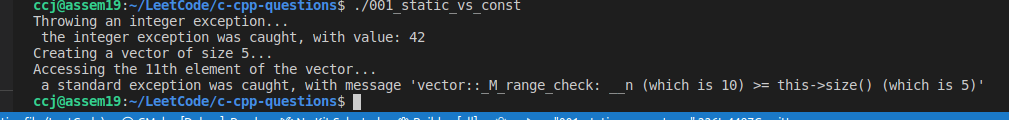### 2) Define New Exceptions

You can define your own exceptions by inheriting and overriding exception class functionality. Following is the example, which shows how you can use std::exception class to implement your own exception in standard way −

#include <iostream>
#include <exception>
using namespace std;

class MyException : public exception {
public:
const char * what () const throw () {
return "C++ Exception";
}
};

int main() {
try {
throw MyException();
} catch(MyException& e) {
std::cout << "MyException caught" << std::endl;
std::cout << e.what() << std::endl;
} catch(std::exception& e) {
//Other errors
}
}

• This would produce the following result −

MyException caught
C++ Exception

• Here, what() is a public method provided by exception class and it has been overridden by all the child exception classes. This returns the cause of an exception.

## 28. C++ Dynamic Memory

A good understanding of how dynamic memory really works in C++ is essential to becoming a good C++ programmer. Memory in your C++ program is divided into two parts −

• The stack − All variables declared inside the function will take up memory from the stack.

• The heap − This is unused memory of the program and can be used to allocate the memory dynamically when program runs.

Many times, you are not aware in advance how much memory you will need to store particular information in a defined variable and the size of required memory can be determined at run time.

You can allocate memory at run time within the heap for the variable of a given type using a special operator in C++ which returns the address of the space allocated. This operator is called new operator.

If you are not in need of dynamically allocated memory anymore, you can use delete operator, which de-allocates memory that was previously allocated by new operator.

### 1) new and delete Operators

There is following generic syntax to use new operator to allocate memory dynamically for any data-type.

new data-type;

Here, data-type could be any built-in data type including an array or any user defined data types include class or structure. Let us start with built-in data types. For example we can define a pointer to type double and then request that the memory be allocated at execution time. We can do this using the new operator with the following statements −

double* pvalue  = NULL; // Pointer initialized with null
pvalue  = new double;   // Request memory for the variable


The memory may not have been allocated successfully, if the free store had been used up. So it is good practice to check if new operator is returning NULL pointer and take appropriate action as below −

double* pvalue  = NULL;
if( !(pvalue  = new double )) {
cout << "Error: out of memory." <<endl;
exit(1);
}


The malloc() function from C, still exists in C++, but it is recommended to avoid using malloc() function. The main advantage of new over malloc() is that new doesn’t just allocate memory, it constructs objects which is prime purpose of C++.

At any point, when you feel a variable that has been dynamically allocated is not anymore required, you can free up the memory that it occupies in the free store with the ‘delete’ operator as follows −

delete pvalue;        // Release memory pointed to by pvalue


### 2) Dynamic Memory Allocation for 1D Arrays

• Consider you want to allocate memory for an array of characters, i.e., string of 20 characters. Using the same syntax what we have used above we can allocate memory dynamically as shown below.

char* pvalue = new char;
double * pVal = new double ;

• To remove the array that we have just created the statement would look like this −

delete [] pvalue; // Delete array pointed to by pvalue
delete [] pVal;


### 3) Dynamic Memory Allocation for multi-dimensional array:

#### 1) Using Single Pointer:

• do 1D index and 2D index conversion via idx = i*rwo_num + j

#include <iostream>
// M x N matrix
#define M 4
#define N 5

// Dynamically Allocate Memory for 2D Array in C++
int main(){
// dynamically allocate memory of size M*N
int * A = new int[M * N];

// assign values to allocated memory
for (int i = 0; i < M; i++)
for (int j = 0; j < N; j++)
*(A + i*N + j) = rand() % 100;

// print the 2D array
for (int i = 0; i < M; i++){
for (int j = 0; j < N; j++)
std::cout << *(A + i*N + j) << " "; // or (A + i*N)[j])

std::cout << std::endl;
}

// deallocate memory
delete[] A;

return 0;
}


#### 2) Using double pointer:

• Please note you CANNOT do this:

int ** A  = new int [ROW_NUM][COL_NUM]; // Wrong!!!

• You will get the following error: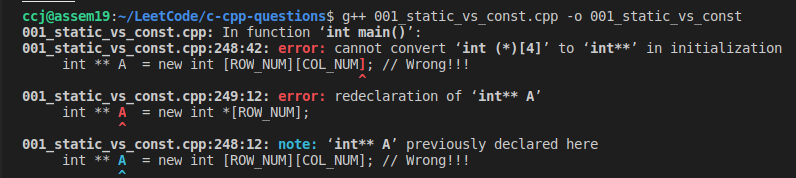int ** ary = new int*[ROW_NUM];
for(int i = 0; i < ROW_NUM; ++i) {
ary[i] = new int[COL_NUM];
}

• The above, for ROW_NUM= 4 and COL_NUM = 5, would produce the following: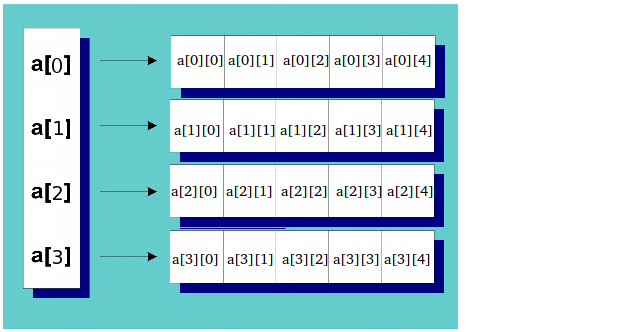• and then clean up would be:

for(int i = 0; i < ROW_NUM; ++i) {
delete [] ary[i];
}
delete [] ary;

• An alternative approach would be to use one large block of memory:

int *ary = new int[ROW_NUM*COL_NUM];
// ary[i][j] is then rewritten as
ary[i*ROW_NUM + j]


### 3) Dynamic Memory Allocation for Objects

Objects are no different from simple data types. For example, consider the following code where we are going to use an array of objects to clarify the concept −

#include <iostream>
using namespace std;

class Box {
public:
Box() {
cout << "Constructor called!" <<endl;
}
~Box() {
cout << "Destructor called!" <<endl;
}
};
int main() {
Box* myBoxArray = new Box;
delete [] myBoxArray; // Delete array

return 0;
}


If you were to allocate an array of four Box objects, the Simple constructor would be called four times and similarly while deleting these objects, destructor will also be called same number of times.

• If we compile and run above code, this would produce the following result: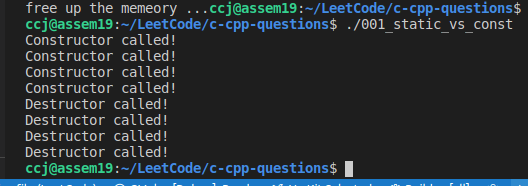## 29. C++ Templates

Templates are the foundation of generic programming, which involves writing code in a way that is independent of any particular type.

A template is a blueprint or formula for creating a generic class or a function. The library containers like iterators and algorithms are examples of generic programming and have been developed using template concept.

There is a single definition of each container, such as vector, but we can define many different kinds of vectors for example, vector <int> or vector <string>.

You can use templates to define functions as well as classes, let us see how they work −

### 1) Function Template

The general form of a template function definition is shown here −

template <class type> ret-type func-name(parameter list) {
// body of function
}


Here, type is a placeholder name for a data type used by the function. This name can be used within the function definition.

The following is the example of a function template that returns the maximum of two values −

template <typename T>
inline T const& Max (T const& a, T const& b) {
return a < b ? b:a;
}

int i = 39;
int j = 20;
cout << "Max(i, j): " << Max(i, j) << endl;

double f1 = 13.5;
double f2 = 20.7;
cout << "Max(f1, f2): " << Max(f1, f2) << endl;


### 2) Class Template

Just as we can define function templates, we can also define class templates. The general form of a generic class declaration is shown here −

template <class type> class class-name {
.
.
.
}


Here, type is the placeholder type name, which will be specified when a class is instantiated. You can define more than one generic data type by using a comma-separated list.

Following is the example to define class Stack<> and implement generic methods to push and pop the elements from the stack −

#include <iostream>
#include <vector>
#include <cstdlib>
#include <string>
#include <stdexcept>

using namespace std;

template <class T>
class Stack {
private:
vector<T> elems;    // elements

public:
void push(T const&);  // push element
void pop();               // pop element

bool empty() const {      // return true if empty.
return elems.empty();
}
};

template <class T>
void Stack<T>::push (T const& elem) {
// append copy of passed element
elems.push_back(elem);
}

template <class T>
void Stack<T>::pop () {
if (elems.empty()) {
throw out_of_range("Stack<>::pop(): empty stack");
}

// remove last element
elems.pop_back();
}

template <class T>
T Stack<T>::top () const {
if (elems.empty()) {
throw out_of_range("Stack<>::top(): empty stack");
}

// return copy of last element
return elems.back();
}

int main() {
try {
Stack<int>         intStack;  // stack of ints
Stack<string> stringStack;    // stack of strings

// manipulate int stack
intStack.push(7);
cout << intStack.top() <<endl;

// manipulate string stack
stringStack.push("hello");
cout << stringStack.top() << std::endl;
stringStack.pop();
stringStack.pop();
} catch (exception const& ex) {
cerr << "Exception: " << ex.what() <<endl;
return -1;
}
}

• If we compile and run above code, this would produce the following result −

7
hello
Exception: Stack<>::pop(): empty stack


## 30. C++ Preprocessor

• The preprocessors are the directives, which give instructions to the compiler to preprocess the information before actual compilation starts.

• All preprocessor directives begin with #, and only white-space characters may appear before a preprocessor directive on a line.

• Preprocessor directives are not C++ statements, so they do not end in a semicolon (;).

There are number of preprocessor directives supported by C++ like #include, #define, #if, #else, #line, etc. Let us see important directives −

### 1) The #define Preprocessor

#define PI 3.14159


### 2) Function-Like Macros

#include <iostream>
using namespace std;

#define MIN(a,b) (((a)<(b)) ? a : b)

int main () {
int i, j;

i = 100;
j = 30;

cout <<"The minimum is " << MIN(i, j) << endl;

return 0;
}


### 3) Conditional Compilation

• #ifndef

#ifndef NULL
#define NULL 0
#endif

• You can compile a program for debugging purpose.

#ifdef DEBUG
cerr <<"Variable x = " << x << endl;
#endif

• You can use #if 0 statement to comment out a portion of the program as follows −

#if 0
code prevented from compiling
#endif
`